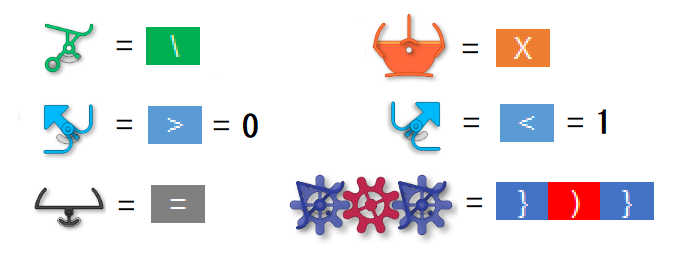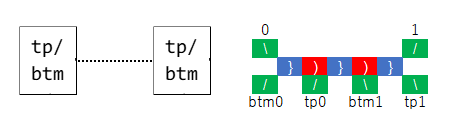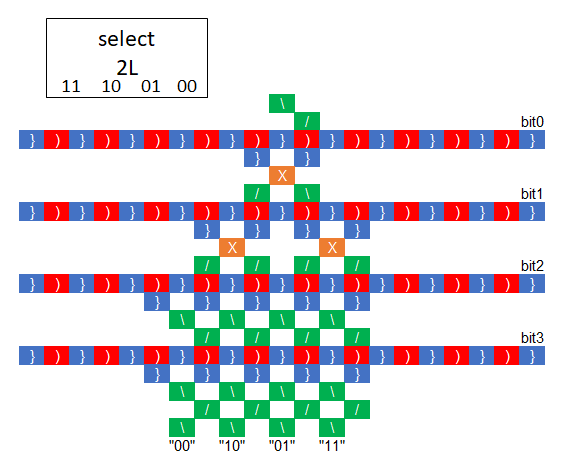# Attempting to build a standard CPU with T.T

There is a CPU called MCPU.
This is a very small but programmable architecture with a program counter.

I have succeeded in reproducing this processor in Turing Tumble.
However, I have reduced the number of bits.
If the number of bits is increased, it can be easily configured in the same way.

``````Mnemon 	Opcode 	Description
NOR 	00AA 	Accu = Accu NOR mem[AA]
ADD 	01AA 	Accu = Accu + mem[AA], update carry
STA 	10AA 	mem[AA] = Accu
JCC 	11DD 	Set PC to DD when carry = 0, clear carry
``````

NoteThe overall diagram is as follows

Indicate each block

top/bottomphase

write

read

selectadd

nor

The operation is briefly described below.

Referring to the BBS(Turing Tumble Difference Engine) and puzzle books(challenge 38, challenge 56), I came up with a way to put the serial information on the ball and transfer it to the memory bit in the upper position.

Carefully examine the structure of the MCPU and move the variables in a total of four separate phases.
For each bit in each phase, divide it into top and bottom. In the top , convert the bits to the color of the ball, and in the bottom, convert the color of the ball to the bits.

The details of each phase are as follows. Pseudo-code is used for explanation.

``````ph1:
imm = mem[pc];
ph2:
pc += 1; route = H(imm);
ph3:
if(route == 00){
buff = mem[L(imm)];
}else if(route == 01){
co = 0; buff = mem[L(imm)];
}else if(route == 10){
buff = acc;
}else if(route == 11){
buff = pc; if(co == 0) buff = imm;
}
ph4:
if(route == 00){
acc = acc NOR buff;
}else if(route == 01){
co += H(acc + buff); acc = L(acc + buff);
}else if(route == 10){
mem[L(imm)] = buff;
}else if(route == 11){
pc = L(buff); co = 0;
}
``````

The ball follows the route in the following order from the first pitch.

phase1.bit0.top
phase1.bit0.bottom
phase1.bit1.top
:
phase1.bit3.bottom
phase2.bit0.top
:
phase4.bit3.bottom

In other words, one instruction is executed for 32 balls.

Yama-chan, this is very impressive. We have talked about making the board expandable, but haven’t come up with a clean way to do it. I would love to see this on a large board someday!